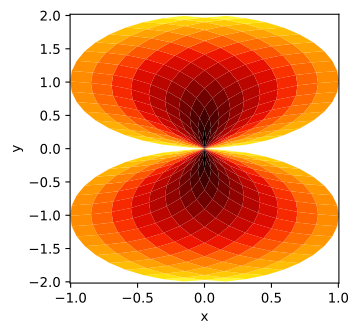Most examples work across multiple plotting backends, this example is also available for:

In :
import numpy as np
import holoviews as hv
hv.extension('matplotlib')
hv.output(fig='svg')## Declaring data¶

In :
n = 20
coords = np.linspace(-1.5, 1.5, n)
X,Y = np.meshgrid(coords, coords);
Qx = np.cos(Y) - np.cos(X)
Qz = np.sin(Y) + np.sin(X)
Z = np.sqrt(X**2 + Y**2)

qmesh = hv.QuadMesh((Qx, Qz, Z))


## Plot¶

In :
qmesh

Out: